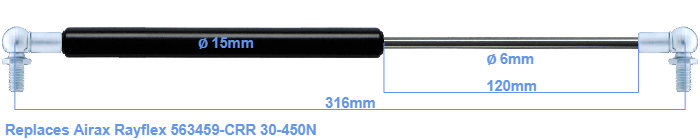# Replacement for Airax Rayflex 563459-CRR 30-450N

\$31.74

Replacement gas spring for the Airax Rayflex 563459-CRR 30-450 Newton. This fits a (possibly already present) ball with a diameter of 10mm. A corresponding ball is included. Brand: HAHN, a Stabilus company.
 Force Choose an option30 Newton40 Newton50 Newton60 Newton80 Newton100 Newton120 Newton140 Newton150 Newton160 Newton180 Newton200 Newton220 Newton240 Newton250 Newton260 Newton280 Newton300 Newton320 Newton340 Newton350 Newton360 Newton380 Newton400 Newton420 Newton440 Newton450 NewtonClear
This gas spring is also known as 5000563459-CRR, 563459-CRR.00.The diameter of the cylinder of this gas spring is 15 millimeters. The rod has a diameter of 6 mm. The rod (the chrome part) is 120 mm long. The total length of this gas strut is 316 mm. Attention: this is the length between the centers of the mounting parts. Without the mounting parts this gas spring is 272 mm long (thread to thread). The force of this replacement gas spring is 30-450 Newton. This is not an official Airax Rayflex gas spring, but a so called replacement. This is a HAHN gas spring. Nevertheless, the dimensions and force are equal.
Category: